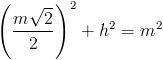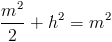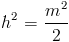## 2/17/10

### Test 1 Section 3 - #19 (page 401)

Disclaimer: I want to point out before we get started that questions like this one are not worth any more than any other question on the test, despite being an order of magnitude more difficult.  Getting this question right is only worth your time if you've been perfect on numbers 1-18.  Otherwise, even if you get this right, all you will have accomplished is earning back points you lost on a much easier problem.  Capiche?

Now, the only way we're going to be able to get at h (the height of our pyramid) is by taking a cross-section of the diagram we've been given.  Picture a razor blade coming down right on the top of it, and slicing it in half right along its corners.  Peep the tracing skillz:

The way we're going to get at h is by using this right triangle (in black):

We already know that e = m (they tell us that in the question), so all we need to use the Pythagorean Theorem is the base.  To figure that out, as we'll usually want to do with 3-D diagrams, we're going to break up the diagram into 2-D bits.  Look at the square that makes up the base of our pyramid (and the line traced by our cross-section):

The red point in the middle is where h hits the floor of our pyramid. Because this is a regular pyramid, it falls right in the center of the square.

Notice what we have here: two 45°-45°-90° triangles, with the red line as the hypotenuse. Each leg (each side of the square) is m.  Using what we know about special triangles (which can be found in the beginning of every math section), we can conclude that the length of the red diagonal is m√2.  Cut that in half, and you have the distance from the corner to the midpoint: (m√2)/2 (shown in black below).
Now go back to our original black triangle (redrawn in 2-D here)...

...time to apply the Pythagorean Theorem.So, our answer is (A).  Phew.